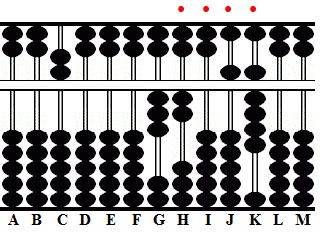###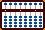Converting Hexadecimal (base 16) to Decimal (base 10)

Traditional methods for converting a hexadecimal number to it's decimal equivalent can be cumbersome and time consuming. I love this method because it is a simple division process which makes it extremely fast and very efficient. The technique requires dividing the hexadecimal number by hex A with the remainder(s) forming the decimal answer.

#### Hex A Division Table (a.k.a. Division rules)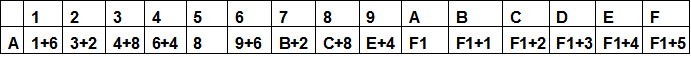Dividing by hex A is remarkably simple in part because the division table for hex A is easily understood. As a result no memorization is necessary. For example look at the rule 4 ÷ A = 6+4; by way of explanation multiply 4 * 16 = 64 and the relationship becomes clear. As we progress through the division table take the following examples: begin by comparing hex B, C and E with their corresponding decimal equivalents in the chart below.

#### Decimal and Hex Equivalents

 DEC 0 1 2 3 4 5 6 7 8 9 10 11 12 13 14 15 16 17 18 19 20 21 22 23 24 25 26 27 28 29 30 31 HEX 0 1 2 3 4 5 6 7 8 9 A B C D E F 10 11 12 13 14 15 16 17 18 19 1A 1B 1C 1D 1E 1FExample 1: Convert hexadecimal 15 to its decimal equivalent. Set the problem with divisor A on the left.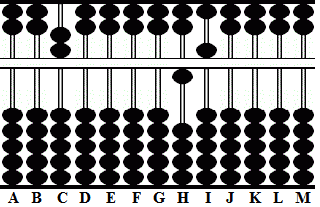Step 1: A meets 1, 1+6, keep 1 on H and add 6 to rod I.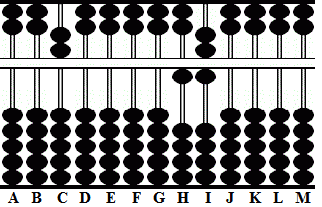Step 2 and the answer: A meets A on rod I, forward 1 to H leaving the answer 21. Hex 15 is decimal 21.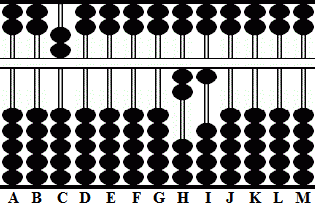Example 2: Convert hexadecimal 9A to its decimal equivalent. Set the problem with divisor A on the left.Step 1: A meets 9, E+4, place E on rod H add 4 to rod I.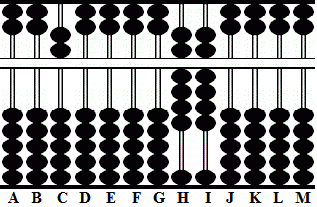Step 2: A meets A on rod H, forward 1 to G.Step 3 and the answer: A meets A on rod I, forward 1 to H leaving the answer 154. Hex 9A is decimal 154.The first two examples were fairly simple. Here's one that is a little more complex.

Example 3: Convert hexadecimal 7D3B to its decimal equivalent.

But before we solve the problem by dividing by A I'd like to show how it's done using the traditional method I most often see on the internet. Comparatively this traditional method is quite a lot of work.

##### Refer to the Decimal and Hex Equivalents Chart; 7D3B = 7, 13, 3, 11

7×16³  +  13×16²  +  3×16¹  +  11×16=
7*4096 + 13*256 + 3*16 + 11*1 =
28672 + 3328 + 48 + 11 = 32059

Now see how simple it is to solve the problem by dividing by hex A. Because of the diagrams and step by step instructions it may seem like a lengthy process. But it really isn't. Once this technique is mastered problems like this can be solved with lightning speed.

Set the problem with divisor A on the left.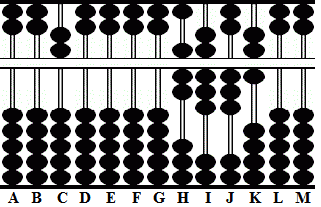Step 1: A meets 7, B+2, place B on rod H add 2 to I. In order to add 2 to rod I use complimentary numbers for 10. Subtract complement 8 and carry 1 to the left. With that carry what was once B on rod H becomes C. Simple abacus basics.```Step 1 A B C D E F G H I J K L M . . . .   0 0 A 0 0 0 0 7 D 3 B 0 0 place B -8 carry +1       0 0 A 0 0 0 0 C 5 3 B 0 0```

Step 2: A meets 5, 8, place 8 on rod I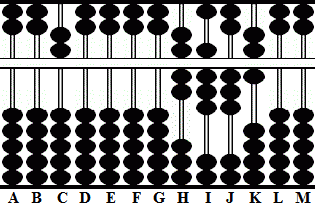Step 3: A meets 3, 4+8, place 4 on J and add 8 to K. In order to add 8 to rod K use complimentary numbers for 10. Subtract complement 2 and carry 1 to the left. With that carry what was 4 on J becomes 5. Again simple abacus basics.

At this point what's left is the integer C85 on rods HIJ and a remainder 9 on rod K signified by the red dot.```Step 3 A B C D E F G H I J K L M . . . .   0 0 A 0 0 0 0 C 8 3 B 0 0 place 4 -2 carry +1    0 0 A 0 0 0 0 C 8 5 9 0 0```

Now divide the integer C85 by A

Step 4: A meets A forward 1, remove A from H and forward 1 to GStep 5: A meets 2, 3+2, place 3 on H and add 2 to I. In order to add 2 to rod I use complimentary numbers for 10. Subtract complement 8 and carry 1 to the left. With that carry what was 3 on H becomes 4. This leaves the integer 140 and the remainder 59 on JK signified by the red dots.```Step 5 A B C D E F G H I J K L M . . . .   0 0 A 0 0 0 1 2 8 5 9 0 0 place 3 -8 carry +1    0 0 A 0 0 0 1 4 0 5 9 0 0```

Now divide the integer 140 by A

Step 6: A meets 1, 1+6, keep 1 on G and add 6 to H. Again use complimentary numbers for 10. Subtract complement 4 and add 1 to the left. This leaves the integer 20 on GH and the remainder 059 as signified by the red dots.```Step 6 A B C D E F G H I J K L M . . . .   0 0 A 0 0 0 1 4 0 5 9 0 0 -4 carry +1    0 0 A 0 0 0 2 0 0 5 9 0 0```

Now divide the integer 20 by A

Step 7 and the answer: A meets 2, 3+2, place 3 on G and add 2 to rod H. With 3 on rod G and remainders on HIJK the answer is 32059. Hex 7D3B is decimal 32059